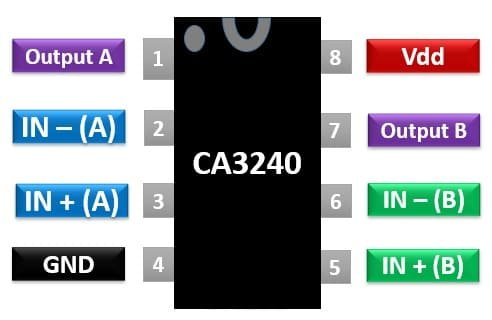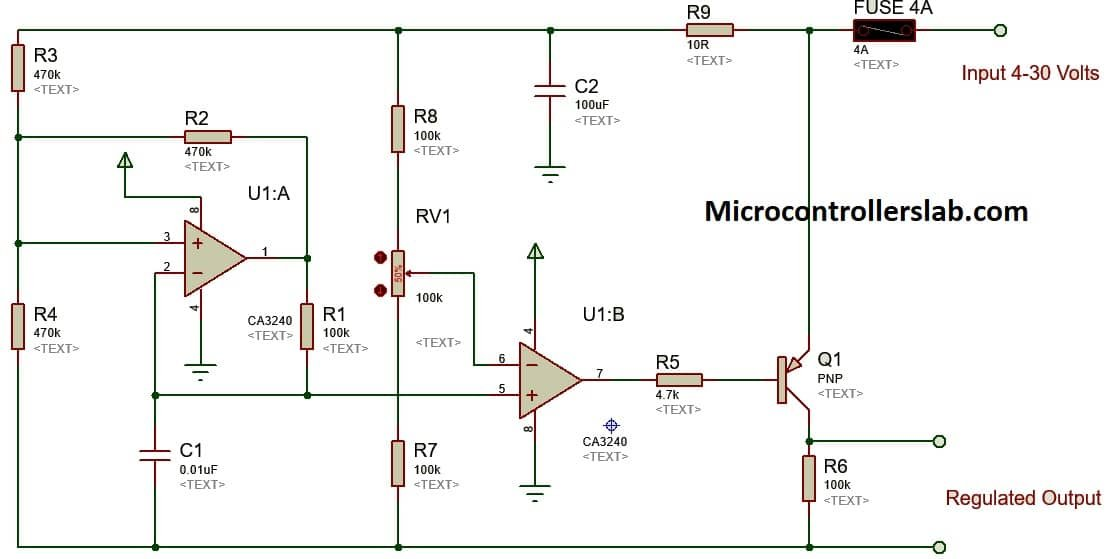# CA3240 BiMOS Op Amp with MOSFET Input/Bipolar Output

The CA3240 IC is an operational amplifier consisting of two op-amps integrated in a single IC. Its internal structure is comprised of both bipolar transistors and Mosfet.  Moreover, it supports Mosfet type input and bipolar output. This P-channel Mosfet in the input circuit allows high impedance and a wide range of common-mode input voltage. These IC’s are internally compensated and used in designing Analogue electronic circuits.

## CA3240 Equivalents and Alternative

This op-amp IC is a dual version of CA3140. The CA3240 is equivalent to TTL based LM741 IC and can replace one another in many applications.

## CA3240 Op-Amp Pinout Diagram

The pinout diagram shows that it has dual outputs for each op-amp. Furthermore, it supports a wide range of operating voltage due to bipolar output transistors.### Pin Configuration Description

• Pin2 and Pin3 are two input pins operational amplifier A. Pin 2 is an inverting i.e., negative pin and pin 3 is a non-inverting i.e., positive pin. Pin 1 is the output pin of this operational amplifier.
• Pin4 and pin8 are the input pins for positive and negative power supply.
• Pins5, 6 and 7 are the inverting, non-inverting and output pin of the operational amplifier B.

## CA3240 Features

• Dual-stage op-amp IC which need a single power supply for its operation.
• Minimum and maximum single voltage supply value is 4V to 36V
• Minimum and Maximum dual voltage supply value is +/- 2 V and +/- 18 V.
• It has a very high impedance () of 5TW and low input current of 10pA due to MOSFET integrated inside IC
• The bandwidth gain product is 4.5MHz and common-mode rejection ratio (CMRR) is 70 dB
• Gate to source protection is provided against static electric discharges.
• CA3240 is a dual version of the CA3140 IC and can replace the TTL based LM741 IC.

## Where to use it?

This IC can be used in comparators, voltage followers, selective inversion circuits and in implementing the designs of transducers amplifiers. Due to is high input impedance, it can be used in designing an accurate circuit for the active filters. Mathematical operations like integration, differentiation, and summing of two values can be performed using this IC. As it is a dual IC, therefore, it can be used to implement two functions together.

## How to use CA3240 Dual Op-Amp IC?

The operation of this IC is simple as you have to only supply input voltages and it will generate the outputs. Due to its extremely high input impedance, it is used in biomedical applications.

### Example Circuit

We can design a DC regulator using CA3240 which can be used in various applications for example to control the brightness of LEDs or speed of a motor/ pump etc. In this circuit, operational amplifier A is applied with a triangular wave signal at its non-inverting input. It acts as a square wave generator.The non-inverting input of the second operational amplifier (B) is connected to a reference signal through a potentiometer which is used to set the trigger point. And opamp B behaves as a comparator. The value of supply voltage should lie between 5 V to 30 V. Greater the supply voltage value, higher will be the efficiency of a regulator. A square wave signal is obtained at pin 7 having a constant frequency and variable pulse width. The circuit diagram of the DC voltage regulator showing the connections and components used is shown below.

### Required Components

QuantityReferencesValue
1C10.01uF
1C2100uF
1FUSE 4A4A
1Q1PNP
5R1, R6-R8, RV1100k
3R2-R4470k
1R54.7k
1R910R
1U1CA3240

## Examples

This section contains example circuits using this operational amplifier IC.

### Differential Light Detector Circuit CA3240

This circuit converts light into voltage using two C30809 photodiodes. Voltage outputs from first stage op-amps are applied to second-stage terminals.## CA3240 Applications

It is used in many projects for implementing different functions. Some of the applications and the projects which can be designed using CA3240 are given below:

• In designing a differential Light detector, CA3240 IC is used for performing a subtracting operation.
• CA3240 is used as a sensing amplifier in designing a dual-level detector
• Photocurrent instrumentation and instrumentation amplifiers are designed through CA3240.
• Multivibrators, Function generators, active filters, constant current or constant voltage amplifiers are all applications of this IC.